search
Search
Unlock 100+ guides
search toc
close
account_circle
Profile
exit_to_app
Sign out
search
keyboard_voice
close
Searching Tips
Search for a recipe:
"Creating a table in MySQL"
Search for an API documentation: "@append"
Search for code: "!dataframe"
Apply a tag filter: "#python"
Useful Shortcuts
/ to open search panel
Esc to close search panel
to navigate between search results
d to clear all current filters
Enter to expand content previewDoc SearchCode Search BetaSORRY NOTHING FOUND!
mic
Start speaking...Voice search is only supported in Safari and Chrome.
Shrink
Navigate to

# Guide on Linear Transformations in Linear Algebra

schedule Aug 12, 2023
Last updated
local_offer
Linear Algebra
Tags
mode_heat
Master the mathematics behind data science with 100+ top-tier guides
Start your free 7-days trial now!
Definition.

# Linear transformations

A linear transformation is a transformation $T:\mathbb{R}^n\to\mathbb{R}^m$ that satisfies the following two properties:

• additive property: $T(\boldsymbol{v}+\boldsymbol{w})=T(\boldsymbol{v})+T(\boldsymbol{w})$ for all $\boldsymbol{v},\boldsymbol{w}\in\mathbb{R}^n$.

• homogeneity property: $T(k\boldsymbol{v})=k\cdot{T(\boldsymbol{v})}$ for all $\boldsymbol{v}\in\mathbb{R}^n$ and $k\in\mathbb{R}$.

Example.

## Functions as linear transformation

Suppose $f:\mathbb{R}\to\mathbb{R}$ is defined by $f(x)=5x$. Show that $f$ is a linear transformation.

Solution. Since functions transform the input $x$ into some output $y$, functions can also be treated as transformations. To prove that a function is a linear transformation, we must check that the function possesses the additive and homogeneity properties.

For any value $x$ and $y$ in $\mathbb{R}$, we have:

\begin{align*} f(x+y) &=5(x+y)\\ &=5x+5y\\ &=f(x)+f(y) \end{align*}

This means that $f$ satisfies the additive property.

We now check for the homogeneity property:

\begin{align*} f(kx) &=5(kx)\\ &=k(5x)\\ &=k\cdot{f(x)}\\ \end{align*}

Since both criteria are satisfied, $f(x)$ is a linear function.

Example.

# Non-linear transformation

Consider the following transformation $T:\mathbb{R}^2\to\mathbb{R}^2$ defined below. Is this a linear transformation?

$$T\left(\begin{pmatrix} x_1\\ x_2\\ \end{pmatrix}\right)= \begin{pmatrix} x_1+x_2\\ x_1+2 \end{pmatrix}$$

Solution. Let's check for the homogeneity property:

\begin{align*} T\left(k\begin{pmatrix} x_1\\ x_2\\ \end{pmatrix}\right)&= T\left(\begin{pmatrix} kx_1\\ kx_2\\ \end{pmatrix}\right)\\ &=\begin{pmatrix} kx_1+kx_2\\ kx_1+2 \end{pmatrix}\\ &\ne{k\begin{pmatrix} x_1+x_2\\ x_1+2 \end{pmatrix}} \end{align*}

Since $T$ does not satisfy the homogeneity property, $T$ is not a linear transformation. Note that the $+2$ term is what makes $T$ non-linear. If the $+2$ was replaced by say $3x_2$, then $T$ would be a linear transformation.

Theorem.

# Linear transformations map the zero vector to the zero vector

If $T:\mathbb{R}^n\to\mathbb{R}^m$ is a linear transformation, then:

$$T(\boldsymbol{0})=\boldsymbol{0}$$

Any transformation that does not satisfy this must be non-linear. Note that the converse is not true, that is, $T(\boldsymbol{0})=\boldsymbol{0}$ does not necessarily imply that $T$ is linear. We will see an example of this shortly.

Proof. If $T$ is a linear transformation, then the homogeneity property must hold for any scalar $k$, that is:

$$T(k\boldsymbol{x})=k\cdot{T(\boldsymbol{0})}$$

Let's set $k=0$ to get:

$$T(\boldsymbol{0})=\boldsymbol{0}$$

This completes the proof.

Example.

## Showing that a transformation is non-linear

Consider the following two functions below. Are they linear transformations?

$$f_1(x)=2x+1,\;\;\;\;\; f_2\left(\begin{pmatrix} x_1\\ x_2\\ \end{pmatrix}\right)= \begin{pmatrix} x_1x_2\\0 \end{pmatrix}$$

Solution. We can use theoremlink to easily check if $f_1$ is non-linear:

$$f_1(0)=2(0)+1\ne0$$

This means that $f_1$ is not linear. This may be mind-blowing 🤯 since we all know that $f_1$ is an equation of a straight line. $f_1$ is indeed a linear equation, but $f_1$ is not considered a linear transformation in the world of linear algebra.

Let's now check the linearity of $f_2$ by first using theoremlink:

$$f_2(\boldsymbol{0})= \begin{pmatrix} 0\\0\\ \end{pmatrix}$$

Unlike $f_1$, we have that $f_2$ satisfies theoremlink. However, we still cannot conclude that $f_2$ is linear - theoremlink only tells us that a transformation is non-linear if $T(0)\ne{0}$. We must check the two defining properties once again - let's check the homogeneity property:

$$f_2\left(\begin{pmatrix}kx_1\\kx_2\\\end{pmatrix}\right)= \begin{pmatrix} k^2x_1x_2\\ 0 \end{pmatrix}\ne{k\begin{pmatrix} x_1x_2\\ 0\\ \end{pmatrix}}$$

Since the homogeneity property is not satisfied, we conclude that $f_2$ is not a linear transformation.

Theorem.

# Linear transformation as matrix-vector product

Let $T:\mathbb{R}^n\to\mathbb{R}^m$ be a linear transformation. $T$ can be expressed as a matrix-vector product:

$$T(\boldsymbol{x})=\boldsymbol{Ax}$$

Where $\boldsymbol{x}$ is a vector in $\mathbb{R}^n$ and $\boldsymbol{A}$ is an $m\times{n}$ matrix.

Proof. Any vector $\boldsymbol{x}$ can be expressed as a linear combination of $n$ standard basis vectors $\boldsymbol{e}_1,\boldsymbol{e}_2,\cdots,\boldsymbol{e}_n$ like so:

\begin{align*} \boldsymbol{x} &=x_1\boldsymbol{e}_1+x_2\boldsymbol{e}_2+\cdots+x_n\boldsymbol{e}_n \end{align*}

Let's apply a linear transformation $T$ on both sides to get:

\begin{align*} T(\boldsymbol{x}) &=T(x_1\boldsymbol{e}_1+x_2\boldsymbol{e}_2+\cdots+x_n\boldsymbol{e}_n)\\ \end{align*}

Since $T$ is a linear transformation, we can use the additive and homogeneity properties to get:

\begin{align*} T(\boldsymbol{v}) &=T(x_1\boldsymbol{e}_1+x_2\boldsymbol{e}_2+\cdots+x_n\boldsymbol{e}_n)\\ &=T(x_1\boldsymbol{e}_1)+T(x_2\boldsymbol{e}_2)+\cdots+T(x_n\boldsymbol{e}_n)\\ &=x_1\cdot{T(\boldsymbol{e}_1)}+x_2\cdot{T(\boldsymbol{e}_2)}+\cdots+x_n\cdot{T(\boldsymbol{e}_n)}\\ &=\begin{pmatrix} \vert&\vert&\cdots&\vert\\ T(\boldsymbol{e}_1)&T(\boldsymbol{e}_2)&\cdots&T(\boldsymbol{e}_n)\\ \vert&\vert&\cdots&\vert\\ \end{pmatrix} \begin{pmatrix}x_1\\x_2\\\vdots\\x_n\end{pmatrix}\\ &=\boldsymbol{Ax} \end{align*}

Here, note the following:

• the second-to-last step used theoremlink to rewrite the linear combination using a matrix-vector product.

• $\boldsymbol{A}$ is an $m\times{n}$ matrix whose columns are the transformed standard basis vectors $T(\boldsymbol{e}_1)$, $T(\boldsymbol{e}_2)$, $\cdots$, $T(\boldsymbol{e}_n)$.

This means we can convert any linear transformation to a matrix-vector product by applying the transformation to the standard basis vectors! This completes the proof.

Example.

## Writing a transformation as a matrix product

Consider the linear transformation $T:\mathbb{R}^2\to\mathbb{R}^3$ below:

$$T\left(\begin{pmatrix} x_1\\ x_2\\ \end{pmatrix}\right)= \begin{pmatrix} 2x_1+3x_2\\ x_1+2x_2\\ 3x_1+x_2\\ \end{pmatrix}$$

Write the transformation in matrix-vector product form.

Solution. The standard basis vectors for $\mathbb{R}^2$ are:

$$\boldsymbol{e}_1= \begin{pmatrix} 1\\0\\ \end{pmatrix},\;\;\;\; \boldsymbol{e}_2= \begin{pmatrix} 0\\1\\ \end{pmatrix}$$

Applying transformation $T$ on these basis vectors yields:

$$T(\boldsymbol{e}_1)= \begin{pmatrix} 2\\1\\3\\ \end{pmatrix},\;\;\;\; T(\boldsymbol{e}_2)= \begin{pmatrix} 3\\2\\1\\ \end{pmatrix}$$

By theoremlink, the linear transformation can be expressed as:

$$T(\boldsymbol{x})= \begin{pmatrix} 2&3\\ 1&2\\ 3&1 \end{pmatrix} \begin{pmatrix} x_1\\ x_2\\ \end{pmatrix}$$

# Geometric interpretation of matrix transformation

Geometrically, a transformation can be interpreted as an operation that changes the position of the input. For instance, consider the following grid: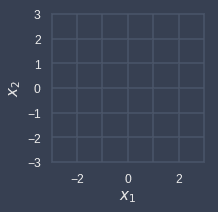Now, consider the following linear transformation:

$$T\left(\begin{pmatrix} x_1\\ x_2\\ \end{pmatrix}\right)= \begin{pmatrix} 2x_1+x_2\\ x_1+3x_2 \end{pmatrix} = \begin{pmatrix} 2&1\\ 1&3 \end{pmatrix} \begin{pmatrix} x_1\\ x_2 \end{pmatrix}$$

By applying this transformation to all the data points over the grid lines, we end up with the following result: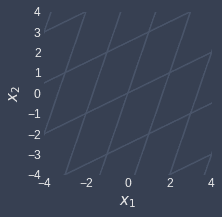Notice how the grid lines are still straight and parallel - this is guaranteed because $T$ is a linear transformation. In contrast, consider a non-linear transformation like so:

$$T\left(\begin{pmatrix} x_1\\ x_2\\ \end{pmatrix}\right)= \begin{pmatrix} \cos(x_1-x_2)\\ \sin(x_1+x_2) \end{pmatrix}$$

Transforming our grid lines will result in non-straight non-parallel lines: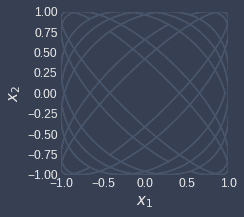Let's now see what happens to the standard basis vectors after a linear transformation:

$$T(\boldsymbol{e}_1)= \begin{pmatrix} {\color{blue}2}&1\\ {\color{blue}1}&3 \end{pmatrix} \begin{pmatrix} 1\\ 0 \end{pmatrix}= \begin{pmatrix} \color{blue}2\\ \color{blue}1 \end{pmatrix},\;\;\;\; T(\boldsymbol{e}_2)= \begin{pmatrix} 2&\color{red}1\\ 1&\color{red}3 \end{pmatrix} \begin{pmatrix} 0\\ 1 \end{pmatrix}= \begin{pmatrix} \color{red}1\\ \color{red}3 \end{pmatrix}$$

Notice how the transformed vectors are actually just the columns of the transformation matrix! Let's define $\boldsymbol{e}'_1$ and $\boldsymbol{e}'_2$ as the transformed standard basis vectors, that is:

• $\boldsymbol{e}'_1=T(\boldsymbol{e}_1)$

• $\boldsymbol{e}'_2=T(\boldsymbol{e}_2)$

Let's visualize the standard basis vectors before and after the transformation:

Standard basis vectors before $T$

Standard basis vectors after $T$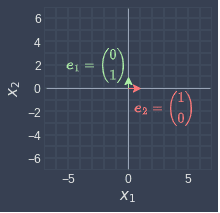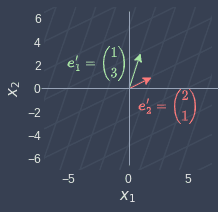Now, suppose we focus on a single point:

$$\boldsymbol{x}= \begin{pmatrix} 2\\ 1\\ \end{pmatrix}$$

Let's visualize this point:

Point before $T$

Point after $T$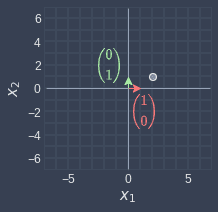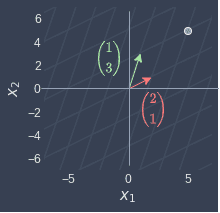Here, we can make the following observations:

• before the transformation - we see that $\boldsymbol{x}$ can be represented using 2 $\color{red}\boldsymbol{e}_1$ and 1 $\color{blue}\boldsymbol{e}_2$.

• after the transformation - we see that $T(\boldsymbol{x})$ can be represented using 2 $\color{red}e_1'$ and 1 $\color{green}\boldsymbol{e}_2'$.

The key here is that the point $\boldsymbol{x}$ is represented using the same linear combinations of the standard basis vectors before and after the transformation.

Let's also verify this mathematically. We can express $\boldsymbol{x}$ using the standard basis vectors:

$$\boldsymbol{x}=\begin{pmatrix} 2\\ 1\\ \end{pmatrix}= 2\begin{pmatrix} 1\\ 0\\ \end{pmatrix}+ 1\begin{pmatrix} 0\\ 1\\ \end{pmatrix}= 2\boldsymbol{e}_1+\boldsymbol{e}_2$$

This tells us that $\boldsymbol{x}$ can be represented using two $\boldsymbol{e}_1$ and one $\boldsymbol{e}_2$.

Let's now transform $\boldsymbol{x}$ to get:

$$T(\boldsymbol{x})= \begin{pmatrix} 2&1\\ 1&3 \end{pmatrix} \begin{pmatrix}2\\1\end{pmatrix}=2 \begin{pmatrix}2\\1\end{pmatrix}+ 1\begin{pmatrix}1\\3\end{pmatrix}= 2{\color{red}\boldsymbol{e}'_1}+{\color{green}\boldsymbol{e}'_2}$$

This tells us $T(\boldsymbol{x})$ can be represented using two $\boldsymbol{e}'_1$ and one $\boldsymbol{e}'_2$.

Example.

## Finding the transformed standard basis vectors

Consider the linear transformation $T:\mathbb{R}^3\to\mathbb{R}^3$ below:

$$T\left(\begin{pmatrix} x_1\\ x_2\\ x_3\\ \end{pmatrix}\right)= \begin{pmatrix} 2&1&7\\ 1&3&1\\ 2&4&5 \end{pmatrix} \begin{pmatrix} x_1\\ x_2\\ x_3 \end{pmatrix}$$

Without doing any computation, derive the transformed standard basis vectors.

Solution. Since we are in $\mathbb{R}^3$, we will have $3$ standard basis vectors $\boldsymbol{e}_1$, $\boldsymbol{e}_2$ and $\boldsymbol{e}_3$. The transformed vectors are simply the columns of the transformation matrix:

$$\boldsymbol{e}'_1=T(\boldsymbol{e}_1)=\begin{pmatrix} 2\\ 1\\ 2\\ \end{pmatrix},\;\;\;\;\; \boldsymbol{e}'_2=T(\boldsymbol{e}_2)=\begin{pmatrix} 1\\ 3\\ 4\\ \end{pmatrix},\;\;\;\;\; \boldsymbol{e}'_3=T(\boldsymbol{e}_3)=\begin{pmatrix} 7\\ 1\\ 5\\ \end{pmatrix}$$
thumb_up
thumb_down
Comment
Citation
Ask a question or leave a feedback...
thumb_up
0
thumb_down
0
chat_bubble_outline
0
settings
Enjoy our search
Hit / to insta-search docs and recipes!